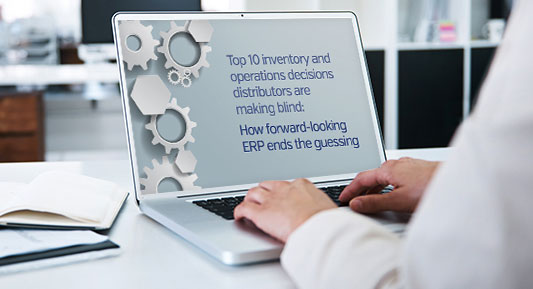# Role Center, KPIs and Cues in Microsoft Dynamics AX 2012

Posted on: August 25, 2016 | By: Jarrod Kraemer | Microsoft Dynamics AX/365

Authored by: Craig Leska – Senior Technical Consultant

The whole purpose of an ERP system is to get information out. You and your coworkers work all day entering sales orders, purchase orders and posting journals. Wouldn’t it be nice if you can display all that information in a Dashboard?

If you have deployed SharePoint in your AX infrastructure then you are in luck, if you have not deployed SharePoint, look at what you are missing.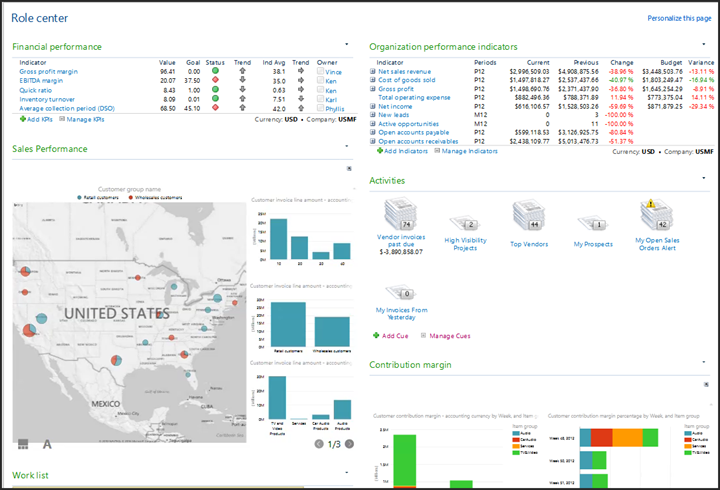The Dashboard, also known as the Home Page displays a wealth of information and in single glance you can take a peek deep inside your organization, you can also create your own KPIs and cues to make your job easier.

Adding the KPIs and cues are an easy thing to do and can be done by someone without a technical knowledge. To add a KPI go to the Home page in Dynamics AX, select Add KPI to a web part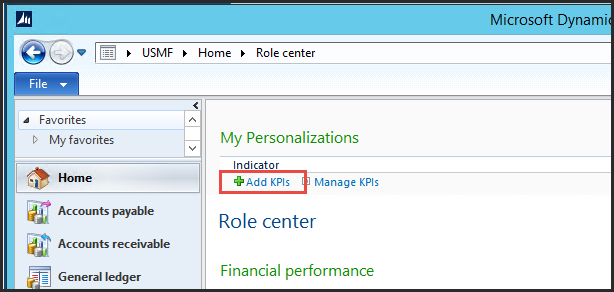We select Active Leads in the Sales and Marketing cube, for a complete list of KPIs see below.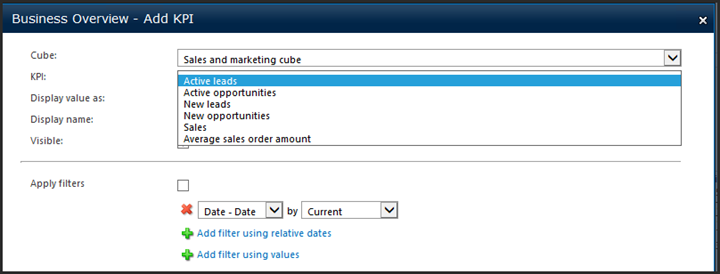On my home page I now have my an Active Leads KPI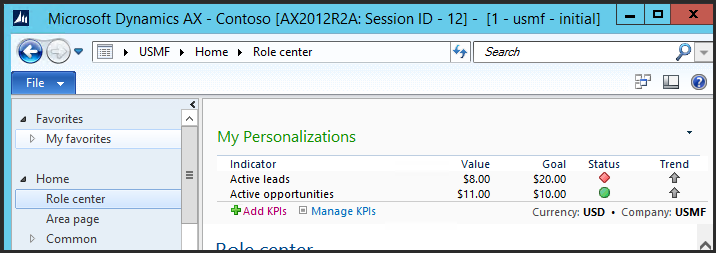You can create Cues from the advance find in any list page. Here is an advance find for “My open invoices from Yesterday.” The key words here are My and Yesterday.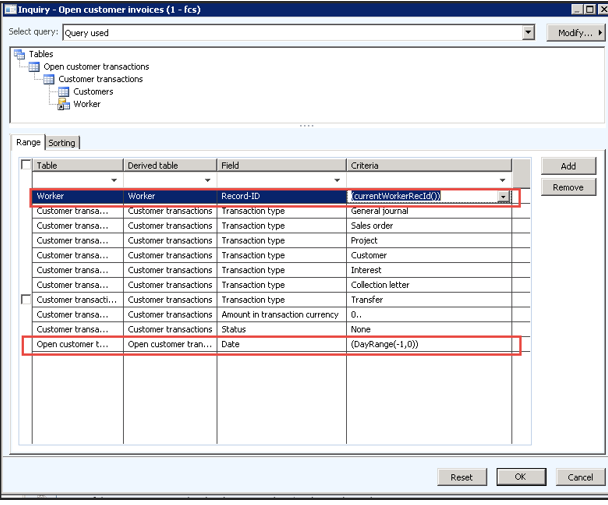To do the My: Create a relation to the worker and add the Record-ID field with the criteria of (currentWorkerRecId()). For the Yesterday, add the date field with the criteria (DayRange(-1,0)). This cue will only display my records from yesterday.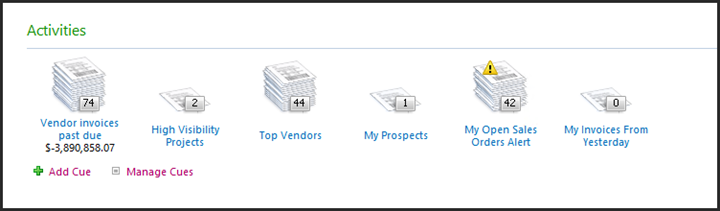From the Activity web part we can add a number of cues or create one from an advanced filter on a list page as the example above. Clicking on a cue will bring us right to those records.

Pretty cool, huh!

Available KPIs in a typical AX 2012 R3 instance.

 Accounts Payable Measure Group Calculation None Accounts Receivable Measure Group Calculation None Budget Control Measure Group Calculation None Budget Plan Measure Group Calculation None Demand Forecast Accuracy Measure Group Calculation None Demand Forecast Measure Group Calculation None Environmental Sustainability Measure Group Calculation Direct energy All The amount of direct energy (such as gasoline or natural gas) consumed by the organization. Indirect energy All The amount of indirect energy (such as electricity or heating services) consumed by the organization. Direct and indirect GHGs All The mass of direct and indirect greenhouse gas emissions produced by the organization’s processes. Other indirect GHGs All The mass of other indirect greenhouse gas emissions (such as employee commuting or business travel) produced by the organization’s processes. Water consumption All The volume of water consumed by the organization. Materials consumption All The mass of materials consumed by the organization. Recycled content All The mass of recycled materials consumed by the organization. Waste water All The volume of waste water produced by the organization. Solid waste All The mass of solid waste produced by the organization. Expense Management Measure Group Calculation Expenses trend Expense lines The percentage change in total expense amount. Policy violations approved per expense report Policy violations log The policy violations log count where action taken = 1 (approved by all approvers), 5 (approved by some approvers). General Ledger Measure Group Calculation Accounts payable turnover* Ledger transactions [Cost of Goods Sold (Reference ID = 35) + (Ending Inventory (Reference ID = 5) for period – Beginning Inventory (Reference ID = 5) for period)] / [Beginning Accounts Payable (Reference ID = 15) + Ending Accounts Payable (Reference ID = 15) / 2]Note * Only the Inventory and Accounts Payable parts of this KPI are accumulated. Accounts receivable turnover* Ledger transactions [Sales (Reference ID = 33) *-1] – Sales Returns and Discounts (Reference ID = 34) / [Beginning Accounts Receivable (Reference ID = 4) + Ending Accounts Receivable (Reference ID = 4) / 2]Note * Only the Accounts Receivable part of this KPI is accumulated. Average days to pay* Ledger transactions Ending Accounts Payable (Reference ID = 15) balance for the period / [Cost of Goods Sold (Reference ID = 35) + (Ending Inventory (Reference ID = 5) for the period – Beginning Inventory (Reference ID=5) for the period)] * Number of days in the periodNote * Only the Accounts Payable and Inventory parts of this KPI are accumulated. Average collection period Ledger transactions (Accounts Receivable (Ref ID 4) / ((Sales (ref id = 33) + Sales returns and discounts (ref id 34)*-1) * number of days in fiscal year to date[ Cash position* Ledger transactions Cash (Reference ID = 1) + Cash Equivalents (Reference ID = 2)Note * Accumulation applies to all calculations. Cash ratio* Ledger transactions Cash Equivalents (Reference ID = 2) + Cash (Reference ID = 1) / (Accounts Payable (Reference ID = 15) + Notes Payable (Reference ID = 16) + Current Maturities on Long Term Debt (Reference ID = 17) + Taxes Payable (Reference ID = 18) + Interest Payable (Reference ID = 19) + Dividends Payable (Reference ID = 20) + Leases Payable (Current) (Reference ID = 21) + Sinking Fund Payable (Current) (Reference ID = 22) + Other Current Liabilities (Reference ID = 23))Note * Accumulation applies to all calculations. Cost of goods sold Ledger transactions Cost of Goods Sold (Reference ID = 35) Current ratio* Ledger transactions ]Cash (Reference ID = 1) + Cash Equivalents (Reference ID = 2) + Short Term Investments (Reference ID = 3) + Accounts Receivable (Reference ID = 4) + Inventory (Reference ID = 5) + Notes Receivables (Reference ID = 6) + Work in Process (Reference ID = 7) + Prepaid Expenses (Reference ID = 8) + Other Current Assets (Reference ID = 9) + Inventory (Reference ID = 5)[ / ]Accounts Payable (Reference ID = 15) + Notes Payable (Reference ID = 16) + Current Maturities on Long Term Debt (Reference ID = 17) + Taxes Payable (Reference ID = 18) + Interest Payable (Reference ID = 19) + Dividends Payable (Reference ID = 20) + Leases Payable (Current) (Reference ID = 21) + Sinking Fund Payable (Current) (Reference ID = 22) + Other Current Liabilities (Reference ID = 23)[Note * Accumulation applies to all calculations. Debt to equity* Ledger transactions Accounts Payable (Reference ID = 15) + Notes Payable (Reference ID = 16) + Current Maturities on Long Term Debt (Reference ID=17) + Taxes Payable (Reference ID = 18) + Interest Payable (Reference ID = 19) + Dividends Payable (Reference ID = 20) + Leases Payable (Current) (Reference ID = 21) + Sinking Fund Payable (Current) (Reference ID = 22) + Other Current Liabilities (Reference ID = 23) + Long Term Debt (Reference ID = 24) / Common Stock (Reference ID = 25) + Preferred Stock (Reference ID = 26) + Additional Paid in Capital: Common (Reference ID= 27) + Additional Paid in Capital: Preferred (Reference ID = 28) + Retained Earnings (Reference ID = 29) + Treasury Stock (Reference ID = 30) + Common Dividends (Reference ID=31) + Preferred Dividends (Reference ID=32) + Encumbrance (Reference ID = 54) + Pre-encumbrance (Reference ID=55)Note * Accumulation applies to all calculations. Debt to total assets* Ledger transactions ]Accounts Payable (Reference ID = 15) + Notes Payable (Reference ID = 16) + Current Maturities on Long Term Debt (Reference ID = 17) + Taxes Payable (Reference ID = 18) + Interest Payable (Reference ID = 19) + Dividends Payable (Reference ID = 20) + Leases Payable (Current) (Reference ID = 21) + Sinking Fund Payable (Current) (Reference ID = 22) + Other Current Liabilities (Reference ID = 23) + Long Term Debt (Reference ID = 24)[ / ]Cash (Reference ID = 1) + Cash Equivalents (Reference ID = 2) + Short Term Investments (Reference ID = 3) + Accounts Receivable (Reference ID = 4) + Inventory (Reference ID = 5) + Notes Receivables (Reference ID = 6) + Work in Process (Reference ID = 7) + Prepaid Expenses (Reference ID = 8) + Other Current Assets (Reference ID = 9) + Long Term Investments (Reference ID = 10) + Property Plant and Equipment (Reference ID = 11) + Accumulated Depreciation (Reference ID = 12) + Intangible Assets (Reference ID = 13) + Other Assets (Reference ID = 14)[Note * Accumulation applies to all calculations. Gross profit Ledger transactions ]Sales (Reference ID = 33)*-1[ – Sales Returns and Discounts Reference ID = 34) – Cost of Goods Sold Gross profit margin Ledger transactions ](Sales (Reference ID = 33) *-1[ – Sales Returns and Discounts (Reference ID = 34) – Cost of Goods Sold Inventory turnover* Ledger transactions Cost of Goods Sold (Reference ID = 35) / ]Beginning Inventory (Reference ID = 5) + Ending Inventory (Reference = 5) / 2[Note * Only the Inventory part of this KPI is accumulated. Net income Ledger transactions Result A – Result B Result A = ]Sales (Reference ID = 33) + Other Income (Reference ID = 48) + Revenues not producing working capital (Reference ID = 50)+ Sales Returns and Discounts (Reference ID = 34)[ *-1 Result B = Cost of Goods Sold (Reference ID = 35) + Selling Expense (Reference ID = 36) + Administrative Expense (Reference ID = 37) + Manufacturing Expense (Reference ID = 38) + Travel and Entertainment Expenses (Reference ID = 39) + Project Operation Expenses (Reference ID = 40) + Salaries Expense (Reference ID = 41 ) + Other Employee Expenses (Reference ID = 42) + Interest Expense (Reference ID = 43) + Tax Expense (Reference ID = 44) + Depreciation Expense (Reference ID = 45) + Income Tax Expense (Reference ID = 46) + Other Expenses (Reference ID = 47) + Charges not using working capital (Reference ID = 49) +Gain or Loss on Asset Disposal (Reference ID = 51) + Amortization of Intangible Assets (Reference ID = 52) Quick ratio* Ledger transactions ]Cash (Reference ID =1) + Cash Equivalents (Reference ID = 2) + Short Term Investments (Reference ID = 3) + Accounts Receivable (Reference ID = 4) + Notes Receivables (Reference ID = 6)[ / (Accounts Payable (Reference ID = 15) + Notes Payable (Reference ID = 16) + Current Maturities on Long Term Debt (Reference ID = 17) + Taxes Payable (Reference ID = 18) + Interest Payable (Reference ID=19) + Dividends Payable (Reference ID = 20) + Leases Payable (Current) (Reference ID=21) + Sinking Fund Payable (Current) (Reference ID = 22) + Other Current Liabilities (Reference ID = 23)Note * Accumulation applies to all calculations. Return on total assets* Ledger transactions (Result A/Result B) X 100 Result A: Net income + Interest expense (Reference ID =43) + Tax expense (Reference ID = 44) + Income tax expense (Reference ID = 46) Result B: Cash (Reference ID = 1) + Cash Equivalents (Reference ID = 2) + Short Term Investments (Reference ID = 3) + Accounts Receivable (Reference ID = 4) + Inventory (Reference ID = 5) + Notes Receivables (Reference ID = 6) + Work in Process (Reference ID = 7) + Prepaid Expenses (Reference ID = 8) + Other Current Assets (Reference ID = 9) + Long Term Investments (Reference ID = 10) + Property Plant and Equipment (Reference ID = 11) + Accumulated Depreciation (Reference ID = 12) + Intangible Assets (Reference ID = 13) + Other Assets (Reference ID = 14) Revenue budget variance Ledger budgets Sum of budget amounts for the period selected for the accounts / Sum of actual balances for revenue accounts for the same time period = Variance. The result is displayed as a percentage. The following ledger account categories are included in the sum of the budget amounts and in the sum of the actual amounts: Sales (Reference ID = 33) + Other Income (Reference ID = 48) + Revenues not producing working capital (Reference ID =50) + Sales Returns and discounts (Reference ID = 34) + Gain/Loss on Asset Disposal (Reference ID = 51)Note If necessary, the amounts for each account category are summed by dimension combination to compare the budget versus actual amounts by department, cost center, and so on. Reference ID 51 is included only if the balance of the accounts in that account category is negative. Otherwise it is excluded. Times interest earned Ledger transactions Result A/ Interest Expense (Reference ID = 43) Result A: Net income + Interest expense (Reference ID =43) + Tax expense (Reference ID = 44) + Income tax expense (Reference ID = 46) Total expenses Ledger transactions Cost of Goods Sold (Reference ID = 35) + Selling Expense (Reference ID = 36) + Administrative Expense (Reference ID = 37) + Manufacturing Expense (Reference ID = 38) + Travel and Entertainment Expense (Reference ID = 39) + Project Operation Expenses (Reference ID = 40) + Salaries Expense (Reference ID = 41 ) + Other Employee Expense (Reference ID = 42) + Interest Expense (Reference ID = 43) + Tax Expense (Reference ID = 44) + Depreciation Expense (Reference ID = 45) + Income Tax Expense (Reference ID = 46) + Other Expenses (Reference ID = 47) + Charges not using working capital (Reference ID =49) + Amortization of Intangible Assets (Reference ID = 52) + Gain/Loss on Asset Disposal (Reference ID = 51)Note Reference ID 51 is included only if the balance of the accounts in that account category is positive. Otherwise it is excluded. Total revenue Ledger transactions ]Sales (Reference ID = 33) + Other Income (Reference ID = 48) + Revenues not producing working capital (Reference ID =50) +Sales Returns and Discounts (Reference ID = 34) + Gain/Loss on Asset Disposal (Reference ID = 51)[ *-1Note Reference ID 51 is included only if the balance of the accounts in that account category is negative. Otherwise it is excluded. Earnings before income tax Ledger transactions ( Sales (ref_id = 33) + Other Income (ref id = 48) + Sales Returns and Discounts (ref id = 34)) *-1 ) – ( COGS (ref id =35) + Selling Expense (ref id = 36) + Admin Expense (ref id = 37) + Manuf Expense (ref id = 38) + T& E Expense (ref id = 39) + Project and Operation Expense (ref id = 40) + Saleries Expense (ref id = 41) + Other Employee Expenses (ref id = 42) + Interst Expense (ref id = 43) + Tax Expense (ref id = 44) + Depreciation Expense (ref id = 45) + Other Expenses (ref id = 47) + Charges not using working capital (ref ID = 49) ) + Revenues not producing working capital (Reference ID=50) + Amortization of intangible assets (ref id = 52) + Gain/loss on asset disposal (refid = 51) Expense budget variance Ledger budgets Sum budget amounts for the: Cost of Goods Sold (refid = 35)+Selling expense (ref id = 36) + Admin exp (refid = 37) + Manu exp (ref id = 38) + Travel and Enter exp (ref id = 39) + Project expenses (ref id = 40) + Salaries exp (ref id = 41 ) + Other emp exp (ref id = 42) + Interest exp (ref id = 43) + Tax exp (ref id = 44) + Depr exp (ref id = 45) + Income tax exp (ref id = 46) + Other expenses (ref id = 47) + Charges not using working capital (Reference ID = 49) + Amortization of intangible assets (ref id = 52) + Gain/loss on asset disposal (refid = 51) / Sum of actual balances for expense accounts for the same time period : Cost of Goods Sold (refid = 35)+Selling expense (ref id = 36) + Admin exp (refid = 37) + Manu exp (ref id = 38) + Travel and Enter exp (ref id = 39) + Project expenses (ref id = 40) + Salaries exp (ref id = 41 ) + Other emp exp (ref id = 42) + Interest exp (ref id = 43) + Tax exp (ref id = 44) + Depr exp (ref id = 45) + Income tax exp (ref id = 46) + Other expenses (ref id = 47) + Charges not using working capital (Reference ID = 49) +Amortization of intangible assets (ref id = 52) + Gain/loss on asset disposal (refid = 51) Note: If necessary, the amounts for each account category are summed by dimension combinationto compare the budget versus actual amounts by department, cost center, and so on. Reference ID51 is included only if the balance of the accounts in that account category is positive. Otherwise it isexcluded.Note Reference ID 51 is included only if the balance of the accounts in that account category is positive. Otherwise it is excluded. Accounts payable Ledger transactions Accounts Payable (Reference ID = 15) Notes payable Ledger transactions Notes Payable (Reference ID = 16) Interest payable Ledger transactions Interest Payable (Reference ID = 19) Other liabilities Ledger transactions Current Maturities on Long Term Debt (Reference ID = 17) + Taxes Payable (Reference ID = 18) + Leases Payable (Current) (Reference ID = 21) + Sinking Fund Payable (Current) (Reference ID = 22) + Other Current Liabilities (Reference ID = 23) + Dividends Payable (Reference ID = 20) Long-term debt Ledger transactions Long Term Debt (Reference ID = 24) Cash and cash equivalents Ledger transactions Cash (Reference ID = 1) + Cash Equivalents (Reference ID = 2) Short-term investment Ledger transactions Short Term Investments (Reference ID = 3) Accounts receivable Ledger transactions Accounts Receivable (Reference ID = 4) Notes receivable Ledger transactions Notes Receivable (Reference ID = 6) Long-term investment Ledger transactions Long Term Investments (Reference ID = 10) Inventory and WIP Ledger transactions Inventory (Reference ID = 5) + Work in progress (Reference ID = 7) Other org assets Ledger transactions Prepaid Expenses (Reference ID = 8) + Other Current Assets (Reference ID = 9) + Property Plant and Equipment (Reference ID = 11) + Accumulated Depreciation (Reference ID = 12) + Intangible Assets (Reference ID = 13) + Other Assets (Reference ID = 14) Days cash on hand Ledger transactions Cash (Reference ID =1) + Cash Equivalents (Reference ID = 2)/]Operating expense – Depreciation expense (Reference ID = 45)/Number of days in period[Note The KPI needs to calculate from the start of the fiscal year through the last completed fiscal time span. Days working capital Ledger transactions ]Working capital X number of days in period[/](Sales (Reference ID = 33) * -1) – Sales Returns and Discounts (Reference ID = 34)Note The KPI needs to calculate from the start of the fiscal year through the last completed fiscal time span. Days inventory on hand Ledger transactions Number of days in period/Inventory turnoverNote The KPI needs to calculate from the start of the fiscal year through the last completed fiscal time span.Note Inventory turnover is defined as: Cost of Goods Sold (Reference ID = 35) / [Beginning Inventory (Reference ID = 5) + Ending Inventory (Reference = 5) / 2]. Inventory turnover needs to likewise calculate from the start of FY thru last completed fiscal time span. Fixed asset turnover Ledger transactions [Sales (Reference ID = 33) *-1) – Sales Returns and Discounts (Reference ID = 34)/Property Plant and Equipment (Reference ID = 11) +Accumulated Depreciation (Reference ID = 12)Note The KPI needs to calculate from the start of the fiscal year through the last completed fiscal time span. EBITDA margin Ledger transactions (EBITDA/](Sales (Reference ID = 33) *-1) – Sales Returns and Discounts (Reference ID = 34))*100Note EBITDA is defined as Net income – Interest Expense(Reference ID = 43) – Tax Expense (Reference ID = 44) -Depreciation Expense (Reference ID = 45) – Income TaxExpense (Reference ID = 46) -Amortization of Intangible Assets (Reference ID = 52) Human Resources Measure Group Calculation None Inventroy Value Measure Group Calculation Days of inventory quantity All Ending quantity / (Net issues quantity / Days to date) Weeks of inventory quantity All Ending quantity / (Net issues quantity / (Days to date / 7)) Months of inventory quantity All Ending quantity / (Net issues quantity / (Days to date / 30)) Inventory turn quantity All Net issues quantity / ((Beginning quantity + Ending quantity) / 2) Days of inventory amount All Ending amount / (Net issues amount / Days to date) Weeks of inventory amount All Ending amount / (Net issues amount / (Days to date / 7)) Months of inventory amount All Ending amount / (Net issues amount / (Days to date / 30)) Inventory turn amount All Net issues amount / ((Beginning amount + Ending amount) / 2) Payroll Measure Group Calculation None Production Measure Group Calculation Production cost Cost calculation This KPI is bound to the Actual vs. planned consumption calculated measure. Production efficiency Production order This KPI is bound to the Production efficiency calculated measure. Profit Tax Measure Group Calculation None Project Accounting Measure Group Calculation Revenue deviation Actuals The difference between actual and forecast revenue. Gross margin deviation Actuals The difference between actual and forecast gross margin. Net WIP deviation Actuals The difference between actual and forecast net work in progress. Cash inflow devation Actuals The difference between actual and forecast cash inflows. Cash outflow deviation Actuals The difference between actual and forecast cash outflows. Net cashflow deviation Actuals The difference between actual and forecast cash inflows less cash outflows. Purchase Measure Group Calculation None Retail Measure Group Calculation Days of inventory quantity All This value is calculated as: Ending quantity / (Net issues quantity / Days to date) Weeks of inventory quantity All This value is calculated as: Ending quantity / (Net issues quantity / (Days to date / 7)) Months of inventory quantity All This value is calculated as: Ending quantity / (Net issues quantity / (Days to date / 30 )) Inventory turn quantity All This value is calculated as: Net issues quantity / ((Beginning quantity + Ending quantity) / 2) Days of inventory amount All This value is calculated as: Ending amount / (Net issues amount / Days to date) Weeks of inventory amount All This value is calculated as: Ending amount / (Net issues amount / (Days to date / 7 )) Months of inventory amount All This value is calculated as: Ending amount / (Net issues amount / (Days to date / 30)) Inventory turn amount All This value is calculated as: Net issues amount / ((Beginning amount + Ending amount) / 2) Total sales All The total net amount. Total returns Retail transaction The amount of total returns. Average ticket size Retail transaction This value is calculated as: Gross amount / Retail transactions count COGS Customer invoice lines The cost of goods sold (COGS) for the particular invoiced item. The COGS value is based on the corresponding inventory transaction. This measure may need an inventory closing where a potential adjustment may occur. Gross margin Customer invoice lines The amount remaining after the cost of goods sold (COGS) has been deducted from the total sales for an item or a given quantity of inventory. Gross margin percentage Customer invoice lines The gross profit margin divided by the total sales revenue, expressed as a percentage. The gross profit margin represents the percent of total sales revenue that a retailer retains after incurring the direct costs associated with producing the goods and services sold. The higher the percentage, the more the retailer retains on each dollar of sales to service its other costs and obligations. Sales by hour Retail transaction lines The average net amount of sales revenue by hour. Inventory turnover Inventory value This value is calculated as: Net issues amount / ((Beginning amount + Ending amount) / 2) GMROII Inventory value Indicates how much gross margin a retailer gets back for each dollar invested in inventory. This value is calculated as: (Sales / Average inventory at cost) * Gross margin percentage Sales per unit area Retail transaction lines The amount of sales per square foot or square meter. Total customers Retail transactions The number of customers. Sales and Marketing Measure Group Calculation Active leads Lead Lead count where Lead Status = 0 (Open). Active opportunities Opportunity Opportunity count where Opportunity Status = 1 (None). New leads Lead Lead count in the past 7 days. New opportunities Opportunity Opportunity count in the past 7 days. Sales Sales order Project and customer invoice sales total. Average sales order amount Sales order Total sales orders (including project) divided by the number of sales orders. Sales Measure Group Calculation None Workflow Measure Group Calculation None

The above list was compiled from https://technet.microsoft.com/en-us/library/jj710378.aspx

For additional information please feel free to reach out to us at info@loganconsulting.com or (312) 345-8817.

All the best!
Logan Consulting
www.loganconsulting.com

25 Brilliant Ideas to Outsmart Your Competition with Microsoft Dynamics### 25 Brilliant Ideas to Outsmart Your Competition with Microsoft Dynamics

Top 10 Inventory & Operations Decisions Distributors Are Making Blind